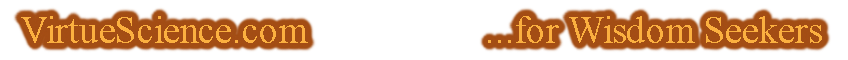Esoteric Wisdom Here are my articles on the Kabbalah, Sacred Geometry and other esoteric subjects. This section needs work and may seem unclear in its present state. Those with a deep curiosity about universal matters should persevere here because there are some very interesting ideas, some of which I have not seen anywhere else.
You are here: Home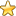The Esoteric Section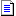# The n-Dimensional Simplexes and Pascal's Triangle

"Number" is not resticted to the 3 dimensions of our physical world but spans all dimensions from zero to infinity. In any dimension there is a shape which constitutes the simplest configuration.

The simplest possible shape in any dimension is called an n-dimensional simplex where n denotes the number of dimensions. For example:

in 0 dimensional space the point is the simplest shape.

in 1 dimensional space the line is the simplest shape.

in 2 dimensional space the triangle is the simplest shape.

in 3 dimensional space the tetrahedon is the simplest shape.

...and so on to infinity. Of course we cannot use a different name for the simplest shape in every dimension so we use the shorthand of n-dimensional simplex.

 n-dimensional simplex English Name 0 Point 1 Line 2 Triangle 3 Tetrahedon 4 Pentalope

 simplex 0 1 2 3 4 5 6 7 0 1 0 0 0 0 0 0 0 1 2 1 0 0 0 0 0 0 2 3 3 1 0 0 0 0 0 3 4 6 4 1 0 0 0 0 4 5 10 10 5 1 0 0 0 5 6 15 20 15 6 1 0 0 6 7 21 35 35 21 7 1 0 7 8 28 56 70 56 28 8 1

This table is a slightly modified Pascal's triangle illustrating a remarkable connection between simplices and simplex figurate numbers.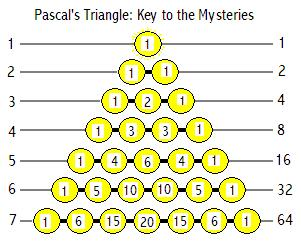The features of a given simplex correspond exactly with a particular level of Pascal's triangle which in turn corresponds with a particular numbers' partition structure.

These partition structures can be represented with binary figures. Base 2 is the simplest possible numerical system of notation. So there is a direct relation between the simplest shape, the simplest base and ways in which a number can be partitioned.

The number of ways in which 5 can be partitioned corresponds with the Tetrahedron.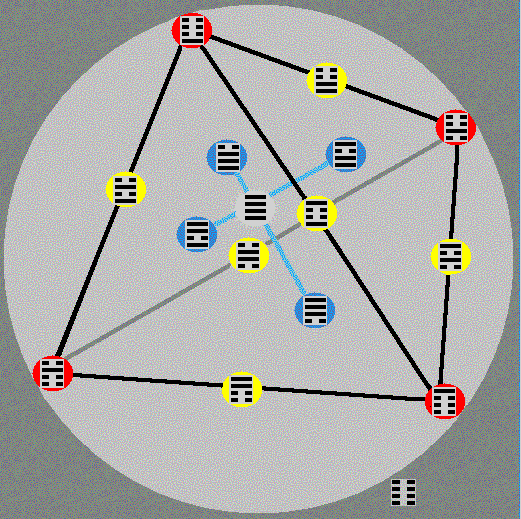You are here: HomeThe Esoteric Section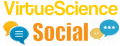Check out the latest Esoteric Wisdom Forum Topics:Hi, I am James Barton an independent truth seeker.
VirtueScience is a unique philosophy and set of tools which I have developed over many years. It combines deep new insights with ancient wisdom.